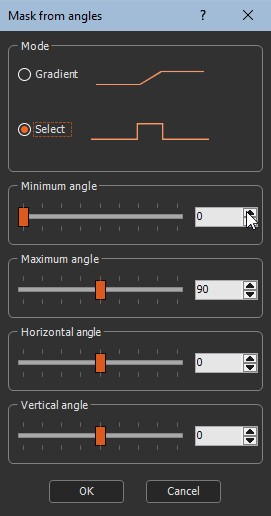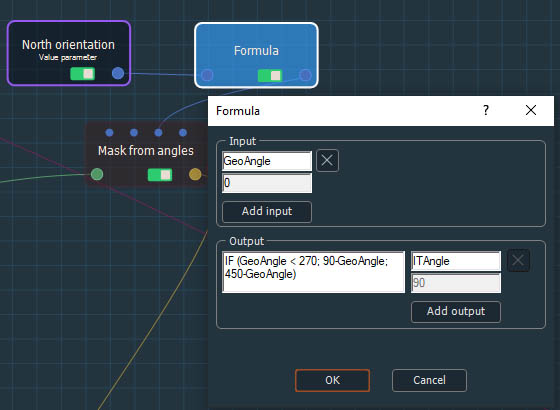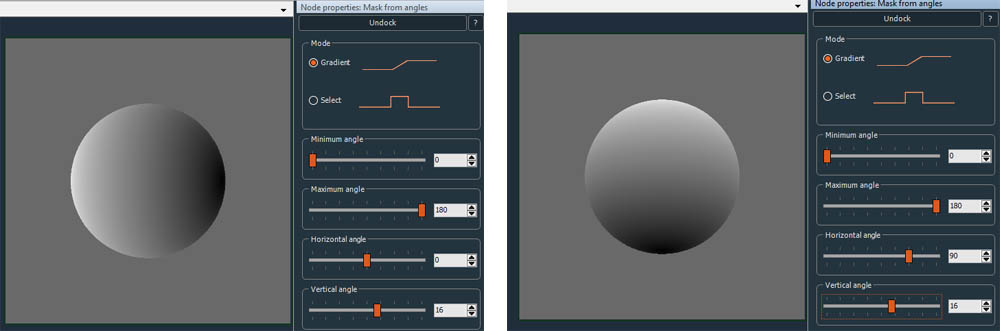This node creates a mask from the angles of a terrain. The mask created has the same size and resolution as the input terrain.

To add a Mask from angles node, right-click in the Graph Editor and select Create Node  > Mask Generation  > Mask from angles.Double click on the node to open its parameters:### Editing a Mask from angles node

#### Minimum and maximum angles

The Minimum and Maximum angles define the interval to select and apply to both modes.
In Gradient mode, values below the minimum angles will be black, and values above the maximum angles will be white.

#### Select mode

At each vertex, the mask has a value of 1 (white color) if the terrain angle is between the Maximum angle and the Minimum angle; otherwise, the mask is set to 0 (black color). The range is set manually.

Horizontal angle corresponds to the angle horizontal to the terrain from -180 degrees to 180 degrees.Result with Horizontal angle = 0 and Vertical angle = 0

To translate a geographic orientation (with 0° = North and 180° = South) into Instant Terra horizontal angle, you can use this formula:

```IF (GeoAngle < 270; 90-GeoAngle; 450-GeoAngle)
```Vertical angle corresponds to the angle vertical to the terrain from -90 degrees to 90 degrees.In this mode, a gradient of values exists, where:

• 0 corresponds to the defined Minimum angle.
• 1 corresponds to the defined Maximum angle.

At each vertex, the mask has a value of 0 (black color) if the angle of the terrain is less than or equal to the minimum terrain angle.
It has a value of 1 (white color) if the terrain angle is higher or equal to the maximum angle.
It has a value between 0 and 1 if the terrain angle lies between these two angles.The gradient interval between black and white corresponds to the part which would be selected (white) in the select mode for the same settings.

### Parameters

Parameter Use
Gradient mode The mask takes a gradient with values between 0 for the minimum angle up to 1 for the maximum angle
Select mode The mask is set to 1 for angles between minimum angle and maximum angle; otherwise it is set to 0
Maximum angle The maximum angle in degrees of the terrain
Minimum angle The minimum angle in degrees of the terrain